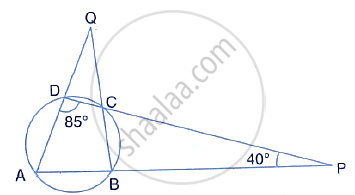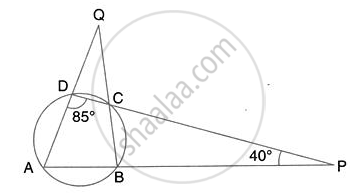Share

# Use the Given Figure to Find: (I) ∠Bad, (Ii) ∠Dqb - Mathematics

Course

#### Question

Use the given figure to find:
(ii) ∠DQB#### Solution(i) By angle sum property of ∆ADP,
∠BAD = 180° - 85° - 40° = 55°

(ii) ∠ABC = 180° - ∠ADC = 180° - 85° = 95°
(pair of opposite angles in a cyclic quadrilateral are supplementary)

By angle sum property,
∠AQB = 180° - 95° - 55°
⇒ ∠DQB = 30°

Is there an error in this question or solution?